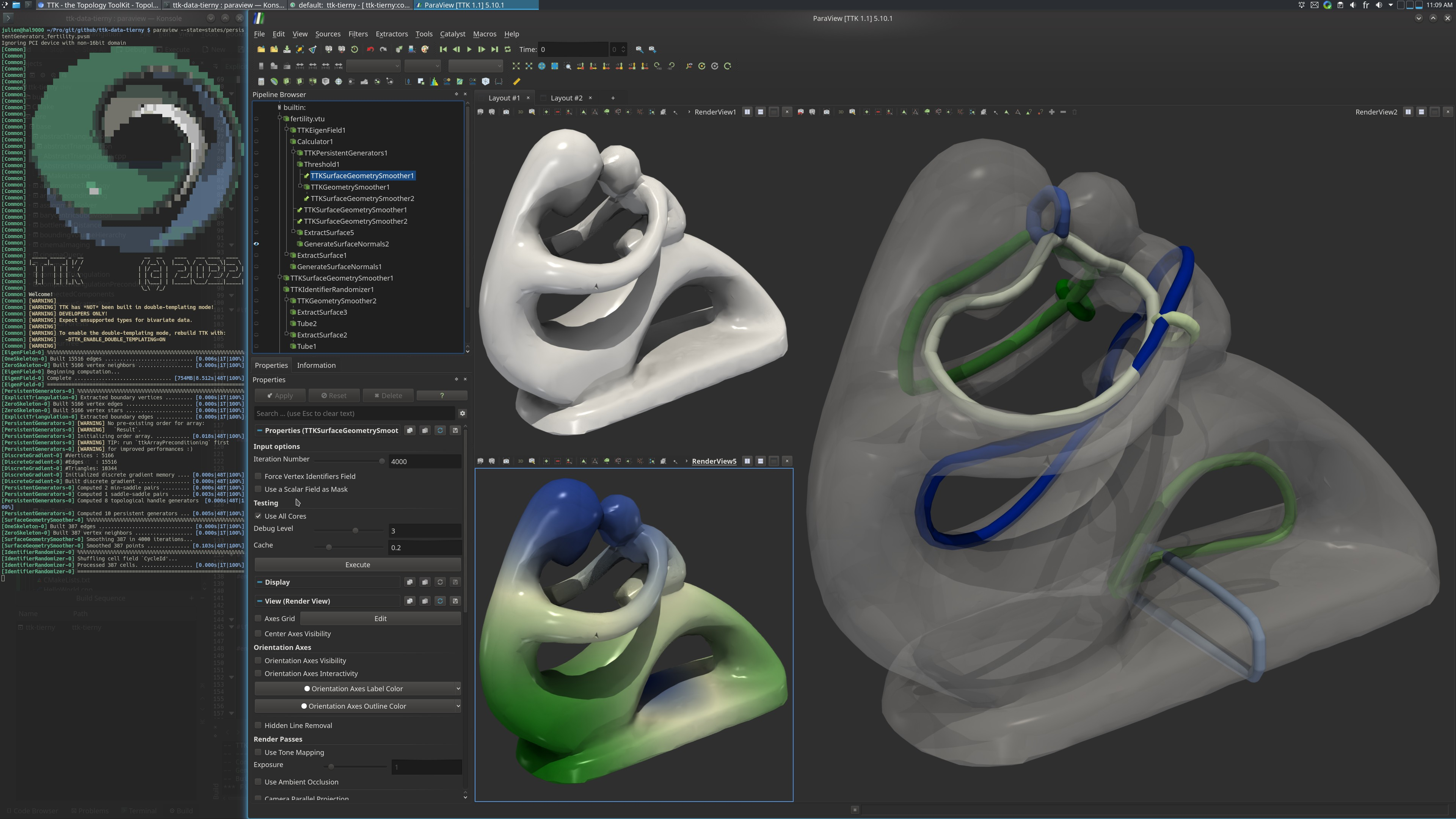# Persistent Generators Fertility¶## Pipeline description¶

This example computes the infinitely persistent 1-cycles of the surface. These cycles smoothly capture the topological handles of the surface.

The surface generators are extracted using the eigenfunctions of the Laplace-Beltrami operator. These eigenfunctions are computed with EigenField Generators are then computed on this smooth scalarfield using PersistentGenerators.

Finally, the cycles are smoothed along the original surface using SurfaceGeometrySmoother

The python script simply computes the cycles and saves the result as a .vtu file.

## ParaView¶

To reproduce the above screenshot, go to your ttk-data directory and enter the following command:

``````paraview states/persistentGenerators_fertility.pvsm
``````

## Python code¶

 ``` 1 2 3 4 5 6 7 8 9 10 11 12 13 14 15 16 17 18 19 20 21 22 23 24 25``` ``````#!/usr/bin/env python from paraview.simple import * # create a new 'XML Unstructured Grid Reader' fertilityvtu = XMLUnstructuredGridReader(FileName=["fertility.vtu"]) # create a new 'TTK EigenField' tTKEigenField1 = TTKEigenField(InputGeometry=fertilityvtu) tTKEigenField1.Numberofeigenfunctions = 100 # create a new 'Calculator' calculator1 = Calculator(Input=tTKEigenField1) calculator1.Function = '"OutputEigenFunctions_4"' # create a new 'TTK PersistentGenerators' tTKPersistentGenerators1 = TTKPersistentGenerators(Input=calculator1) tTKPersistentGenerators1.ScalarField = ["POINTS", "Result"] tTKPersistentGenerators1.PruneHandlesGenerators = 1 # create a new 'Threshold' threshold1 = Threshold(Input=tTKPersistentGenerators1) threshold1.Scalars = ["CELLS", "IsFinite"] SaveData("PersistentGeneratorsFertility.vtu", threshold1) ``````

To run the above Python script, go to your ttk-data directory and enter the following command:

``````pvpython python/persistentGenerators_fertility.py
``````

## Inputs¶

• fertility.vtu: A 2-dimensionnal triangulation of the fertility dataset.

## Outputs¶

• `PersistentGeneratorsFertility.vtu`: the infinitely persistent 1-cycles of the surface.

## C++/Python API¶

EigenField

PersistentGenerators

SurfaceGeometrySmoother